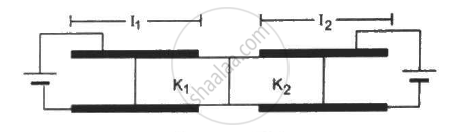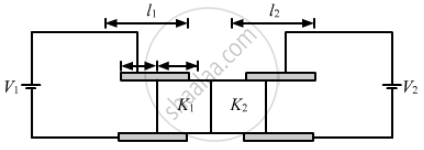Department of Pre-University Education, KarnatakaPUC Karnataka Science Class 12
Share

# Figure Shows Two Parallel Plate Capacitors with Fixed Plates and Connected to Two Batteries. the Separation Between the Plates is the Same for the Two Capacitors. the Plates Are Rectangular - Physics

ConceptCapacitors and Capacitance

#### Question

Figure shows two parallel plate capacitors with fixed plates and connected to two batteries. The separation between the plates is the same for the two capacitors. The plates are rectangular in shape with width b and lengths l1 and l2. The left half of the dielectric slab has a dielectric constant K1 and the right half K2. Neglecting any friction, find the ration of the emf of the left battery to that of the right battery for which the dielectric slab may remain in equilibrium.#### Solution

Let the potential of the battery connected to the left capacitor be V1 and that of the battery connected to the right capacitor be V2.

Considering the left capacitor,

Let the length of the part of the slab inside the capacitor be x.

The left capacitor can be considered to be two capacitors in parallel.

The capacitances of the two capacitors in parallel are :-

C_1 = (K_1∈_0bx)/d , C_2 = (∈_0b(l_1 - x))/d

C1 is the part of the capacitor having the dielectric inserted in it and C2 is the capacitance of the part of the capacitor without dielectric.

As, C1 and C2 are in parallel.

Therefore, the net capacitance is given by

C = C_1 + C_2

C = (K_1∈_0bx)/d + (∈_0b(l_1 - x))/d

C = (∈_0b)/d [l_1 + x(K_1 - 1)]

Therefore, the potential energy stored in the left capacitor will be

U = 1/2CV_1^2

U = (∈_0bV_1^2)/(2d)[l_1 + x(K_1 - 1)]  ..........(1)

The dielectric slab is attracted by the electric field of the capacitor and applies a force in left direction.

Let us consider electric force of magnitude F pulls the slab in left direction.

Let there be an infinitesimal displacement dx in left direction by the force F.

The work done by the force = F.dxLet us consider a small displacement dx of the slab in the inward direction. The capacitance will increase, therefore the energy of the capacitor will also increase. In order to maintain constant voltage, the battery will supply extra charges, therefore the battery will do work.

Work done by the battery = change in energy of capacitor + work done by the force F on the capacitor

dW_B = dU + dW_F

Let the charge dq is supplied by the battery, and the change in the capacitor be dC

dW_B = (dq).V = (dC). V^2

dU = 1/2(dC).V^2

(dC).V^2 = 1/2(dC).V^2 + F.dx

1/2(dC).V^2 = F.dx

⇒ F =  1/2 (dC)/dxV_1^2

⇒ F = 1/2d/dx ((∈_0b)/d[l_1 + x(K_1 - 1)])V_1^2

⇒ F = (∈_0bV_1^2(K_1 - 1))/(2d)

⇒ V_1^2 = (F xx 2d)/(∈_0b(K_1 - 1)) ⇒ V_1 = sqrt ((F xx 2d)/(∈_0b(K_1 - 1)))

Similarly, for the right side the voltage of the battery is given by

V_2 = sqrt ((F xx 2d)/(∈_0b(K_2 - 1)))

Thus , the ratio of the voltages is given by

V_1/V_2 = sqrt ((F xx 2d)/(∈_0b(K_1 - 1)))/sqrt ((F xx 2d)/(∈_0b(K_2 - 1))

⇒ V_1/V_2 = (sqrt(K_2 -1))/(sqrt(K_1 - 1))

Thus, the ratio of the emfs of the left battery to the right battery is V_1/V_2 = (sqrt(K_2 -1))/(sqrt(K_1 - 1))

Is there an error in this question or solution?

#### Video TutorialsVIEW ALL 

Solution Figure Shows Two Parallel Plate Capacitors with Fixed Plates and Connected to Two Batteries. the Separation Between the Plates is the Same for the Two Capacitors. the Plates Are Rectangular Concept: Capacitors and Capacitance.
S